Conic Sections Basics Chapter Exam

Exam Instructions:

Choose your answers to the questions and click 'Next' to see the next set of questions. You can skip questions if you would like and come back to them later with the yellow "Go To First Skipped Question" button. When you have completed the practice exam, a green submit button will appear. Click it to see your results. Good luck!

Page 1

Question 2 2. Which type of conic section is shown below?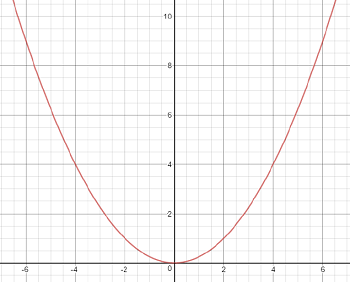Question 3 3. What is the radius of the circle described by the following equation?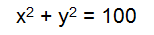Question 4 4. Which conic section is described by the equation shown below?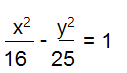Question 5 5. What is the length of the semi-major axis in the ellipse that is described by the following equation?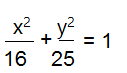Page 2

Question 6 6. What is the eccentricity (to two decimal places) of the conic section represented by this equation?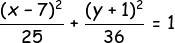Question 7 7. What is the eccentricity (to two decimal places) of the conic section represented by this equation?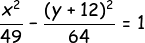Question 8 8. What is the eccentricity (to two decimal places) of the conic section represented by this equation?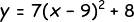Question 10 10. What is the eccentricity (to two decimal places) of the conic section represented by this equation?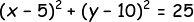Page 3

Question 12 12. Which conic section is this?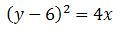Question 13 13. Which conic section is this?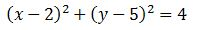Question 15 15. Which conic section is this?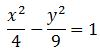Conic Sections Basics Chapter Exam Instructions

Choose your answers to the questions and click 'Next' to see the next set of questions. You can skip questions if you would like and come back to them later with the yellow "Go To First Skipped Question" button. When you have completed the practice exam, a green submit button will appear. Click it to see your results. Good luck!

Support